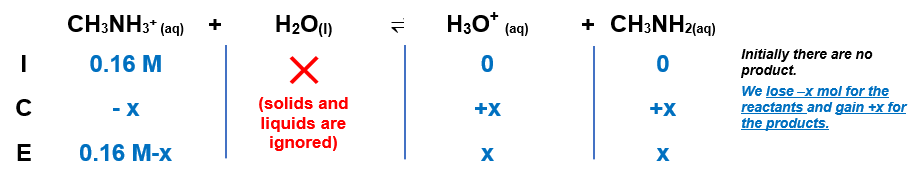# Problem: Solve an equilibrium problem (using an ICE table) to calculate the pH of each of the following solutions.0.16 M CH3 NH3 Cl

###### FREE Expert Solution

We’re being asked to calculate the pH of a 0.16 M CH3NH3Cl using an ICE chart.

CH3NH3+ is a conjugate acid of the base CH3NH2. We need to use the Kb for CH3NH2 to find the Ka of its conjugate acid, CH3NH3+. Let us just omit Clsince it is neutral.

We can find on books and the internet that the Kb of CH3NH2 is 4.4x10-4. Therefore, the Ka of CH3NH3+ is:

Ka = 2.27x10-11

Since CH3NH3+ has a very low Ka value, it’s a weak acid. Remember that weak acids partially dissociate in water and that acids donate H+ to the base (water in this case). The dissociation of CH3NH3+ is as follows:

CH3NH3+(aq) + H2O(l)  H3O+(aq) + CH3NH2(aq); Ka = 2.27×10–11

From this, we can construct an ICE table. Remember that liquids are ignored in the ICE table.The Ka expression for CH3NH3+ is:

$\overline{){{\mathbf{K}}}_{{\mathbf{a}}}{\mathbf{=}}\frac{\left[{H}_{3}{O}^{+}\right]\left[{\mathrm{CH}}_{3}{\mathrm{NH}}_{2}\right]}{\left[{\mathrm{CH}}_{3}{{\mathrm{NH}}_{3}}^{+}\right]}}$

Liquids are ignored in the Ka expression.

Note that each concentration is raised by the stoichiometric coefficient: [CH3NH3+], [H3O+] and [CH3NH2] are raised to 1.

86% (140 ratings)###### Problem Details

Solve an equilibrium problem (using an ICE table) to calculate the pH of each of the following solutions.

0.16 M CH3 NH3 Cl